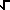# Math2.org Math Tables: Complexity(Math)

### Rationalle that 1/i = -i

We know that by definition
i =( -1 )
Similarly,
i*i =( -1 ) *( -1 ) =( -1 )2 = -1
by some algebra we get
i*i = -1
-i*i = 1     (multiply both sides by -1)
-i = 1/i     (divide both sides by i)